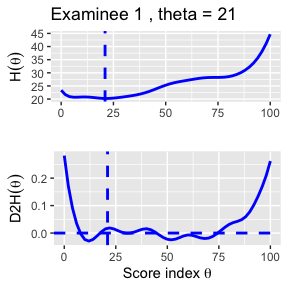# Quantshort

#### 12/19/2022

##  "/private/var/folders/98/j_0ystlj20112y6vtrxz5gk00000gp/T/RtmpIAXlCN/Rinstcd61085af36/TestGardener"
library(TestGardener)
#
# Attaching package: 'fda'
# The following object is masked from 'package:graphics':
#
#     matplot
# Loading required package: rgl

# Introduction

In this vignette we run through an analysis of examination data selected from the SweSAT Quantitative subtest that was analyzed in our previous publications and in our manual for TestGardener. We will use only the 24 questions in the SweSAT that were designed to test the examinee’s ability to make mathematical calculations. We also selected at random only 1000 of the roughly 53,000 examinees who took the test.

The test was administered in the fall of 2013, and required both a morning and afternoon session to complete. Forty items were presented in each session. Questions 23 to 28 and 63 to 68 had five options as answers, and the remainder had only four. In addition to these options, there was an additional last “answer” for each question for a failure to respond to the question or illegal or illegible answers, a choice that is often described as “missing responses. The number of such responses is low in this test, but must will be treated as a response in order to ensure that probabilities of choice for each question add to one.

The questions are organized into four groups according to the kind of mathematical thinking required. The first 12 are mathematical problem solving questions, involving purely mathematical calculations. The next 10 require quantitative comparisons where a typical set of options are “I is larger than II”, “II is larger than I”, I is equal to II”, and “Information is insufficient.” The next 6 involve quantitative reasoning and have five options. The final 12 require extracting information from diagrams, tables and maps. The questions and their answers are available in “.pdf” files within same “Vignettes” folder as this code and text “.Rmd” file.

The goal here is to make a more detailed description of the interactions of the 5000 examinees with the 80 test items than was carried out with the 24 item version of this test.

There are five distinct stages to the processing of the data: 1. Reading in the data and selecting the portion to analyze. 2. Using the data to set up a named list object containing the information essential to their analysis. 3. The actual analysis. 4. Producing graphs and other displays for evaluation of the performance of the test questions. 5. Describing aspects of the interactions between item and examinees. 5. Simulating data in order to assess the accuracy of estimated quantities.

Here the data required for the analysis are entered. There are up to five objects to enter:
- a title for the analysis - the choice data for the 5000 examinees - the right answer key - a character string for each item or question to display in plots - a character string for each option choice within each item The two essential inputs are the choice data and the right answer key.

The choice data are an N by n matrix called U_5000 in the code. The rows of U_5000 correspond to examinees and the 80 columns to items or questions. Each number in U_5000 is the index of the option or answer that the examinee has chosen using the number 1 to M where M is the number of answers available for choice. We call this data structure index mode data, in contrast to score data where the number indicate pre-assigned scoring weights.

The choice indices may also be stored in a dataframe where all variables are of the factor type. We have avoided this level of sophistication since the only operations on U_5000 are summing and retrieving values. Given the potential size of the choice data, it can be worthwhile to place additional restrictions on the structure such being in integer mode.

The second data to read in are the indices of the right answers in the vector key. This object is used to assign scores of 0 to wrong answers and 1 to right answers.

The reader may find it helpful to consult man pages for the functions used using the command help("filename") to obtain more detail and see example code.

First we will set up a title for the data to be used in plots:

titlestr  <- "SweSAT-Q: 24 math analysis items and 1000 examinees"

Then we set up the path or connection to the location of the choice data.

The first five lines in the file “U_short_5000.txt” in TestGardener’s data folder are:

21323211244334312213413543333122422234448412231413431343313231533254433231121343 21223212144334242213413353433112123234441412221413411343113133513241441121231343 21423433234314141333433421444212314214443413231423432412313431332154123134242343 21344243214214312314223525223111142314324132124231431243313113534233123134444123 22143311341414332244143553222231121231431414343424432342313233555231143223121143

We use the basic scan()’ command to read the 1000 lines in the file like this:

U <- scan("U_5000.txt","o")
N <- length(U) # Number of examinees

The command assumes that the working directory is the TestGardener folder, within which there is a “data” folder containing various data files. The second argument deals with the fact that there is no space between values, and the last argument declares each symbol to be a character value. The data are now 1000 strings, each 24 characters long, and the second line sets N, the number of examinees.

We will use the stringr package to break the each 80 character string into separate characters and then convert these characters to integers. The result is an integer vector of length 80 x 5000 = 40,000. The number of questions n is this length divided by the number of examinees. The third line reformats Uvector into an N by n matrix.

Uvector <- as.integer(unlist(stringr::str_split(U,"")))
n       <- length(Uvector)/N # Number of items
U       <- matrix(Uvector,N,n,byrow=TRUE)

However, we have also set up both U and key objects as .rda compressed files that are automatically loaded if library(TestGardener) has been entered.

There are many other ways in R to achieve the same result, of course, and this is unlikely to be viewed as the most elegant.

Inputting the right answer key object uses the same techniques, and we have in fact saved these also as a set of integer index values looking like the data themselves.

key   <- scan("key_5000.txt","o")
key <- as.integer(unlist(stringr::str_split(key,"")))

## Selecting the 24 mathematical problem solving items and the subsample of 1000 examinees

itemindex <- c(1:12, 41:52)
examinees <- 4001:5000
U <- U[examinees,itemindex]
key <- key[itemindex]
N <- 1000
n <- 24

## Setting up the analysis

Now we turn to computing objects using these data that are essential to the analysis of the data.

All questions for these 24 questions in the test have four answers, but we will add an additional category for answers that are missing or illegitimate.

noption <- rep(5,n)

The data have two integers for missing or illegitimate items: 8 and 9. We change these to the garbage category 5.

for (j in 1:N) {
for (i in 1:n) {
if (U[j,i] == 8 || U[j,i] == 9) U[j,i] = 5
}
}

Now we turn to code that sets up objects that are essential to the analysis but that the analyst may want to set up using code rather than inputting. The first of these is a list vector of length n where each member contains a numeric vector of length M containing the designed scores assigned to each option. For multiple choice tests, that is easily done using the following code:

ScoreList <- list() # option scores
for (item in 1:n){
scorei <- rep(0,noption[item])
scorei[key[item]] <- 1
ScoreList[[item]] <- scorei
}

There is also the possibility of inputting strings for each question and each option within each question. We won’t use this feature in this analysis.

Next, we set up a named list called optList that contains ScoreList and default values for the item and option strings:

optList <- list(itemLab=NULL, optLab=NULL, optScr=ScoreList)

We’re now finished with the data input and setup phase. From these objects we have a function make_dataList that completes the set up:

Math_dataList <- TestGardener::make.dataList(U, key, optList)
names(Math_dataList)
#   "U"           "N"           "n"           "titlestr"    "optList"
#   "WfdList"     "key"         "grbg"        "WfdPar"      "noption"
#  "nbin"        "scrrng"      "scrfine"     "scrvec"      "scrjit"
#  "itmvec"      "percntrnk"   "thetaQnt"    "Wdim"        "PcntMarkers"
#  "titlestr"    "grbg"

The result is a named list with many members whose values may be required in the analysis phase. Consult the documentation using help("make.dataList.R").

One default option requires a bit of explanation. Function make.dataList() computes sum scores for each examinee, which in the multiple choice case are simply counts of the number of correct answer choices. The analysis phase does not use these sum scores directly. Instead, it uses the rank orders of the sum scores (ordered from lowest to highest) divided by N and multipled by 100, so that the scores now look like percentages, and we refer to them as “percent ranks.”

A problem is that many examinees will get the same rank value if we order these ranks directly. We can’t know whether there might be some source of bias in how these tied ranks are arranged. For example, suppose male examinees for each rank value are followed by female examinees with that rank value. We deal with this problem by default by adding a small random normally distributed number to each tied rank that is too small to upset the original rank order. This within-rank randomization is called “jittering” in the statistics community, and its tends to break up the influence of any source of bias. This is the default, but can be turned off if desired.

These jittered percent ranks are used to provide initial values for a cycled optimization procedure used by TestGardener.

Let’s make our first plot a display of the histogram and the probability density function for the sum scores.

hist(Math_dataList$scrvec, Math_dataList$scrrng, xlab="Sum Score",
main=titlestr)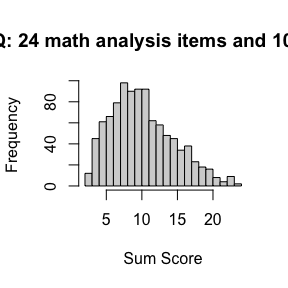This histogram tells us a number of things. First, this is obviously a difficult test from the standpoint of the examinees at or below the mid-score of 40 questions correct. It is even difficult for the strongest examinees since no examinees obtained perfect scores. But the fact that no examinees had a sum score less tan 10 needs explaining, too. When completely lost, an examinee can always guess and choose one at random. For four-option question, this will strategy will produce a correct answer about 20 times and, for a test this long, is unlikely to produce a score less than 10. Guessing inflates sum scores over what we would desire, and our analyses will compensate for this by recognizing the signs of guessing and deflating the resulting score.

The next steps are optional. The analysis can proceed without them, but the analyst may want to look at preliminary results that are associated with the the objects in the set up phase.

First we compute item response or item characteristic curves for each response within each question. These curves are historically probability curves, but we much prefer to work with a transformation of probability, “surprisal = - log(probability),” for two reasons: (1) this greatly aids the speed and accuracy of computing the curves since they are positive unbounded values, and (2) surprisal is in fact a measure of information, and as such has the same properties as other scientific measures. It’s unit is the “bit” and what is especially important is that any fixed difference between two bit values means exactly the same thing everywhere on a surprisal curve.

The initial surprisal curves are achieved as follows using the important function Wbinsmth():

theta     <- Math_dataList$percntrnk thetaQnt <- Math_dataList$thetaQnt
WfdResult <- TestGardener::Wbinsmth(theta, Math_dataList)

One may well want to plot these initial surprisal curves in terms of both probability and surprisal, this is achieved in this way:

WfdList <- WfdResult$WfdList binctr <- WfdResult$aves
Qvec    <- c(5,25,50,75,95)
indfine <- seq(0,100,len=101)
TestGardener::ICC.plot(indfine, WfdList, Math_dataList, Qvec, binctr,
Wrng=c(0,3), plotindex=1)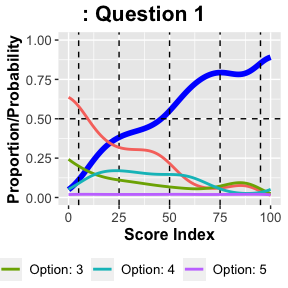Here Ωand elsewhere we only plot the probability and surprisal curves for the first time in order to allow this vignette to be displayed compactly. There are comments on how to interpret these plots in the call to function ICC.plot() after the analysis step.

The bottom plot displays surprisal, the logarithm to the base M of the negative of probability. Surprisal is a valuable transform of probability. As the name suggests, when probability approach one, surprisal move close to zero, but probabilities near zero imply surprisals high surprisals. Surprisal is a measure if information having the “M-bit” as its unit. Most of us are familiar with the “2-bit”, associated with coin tosses. Surprisal is essentially the number of heads you would have to toss on the average to yield a particular probability. Probability 0.5 corresponds to 1 “2-bit”, and the famous probabilities 0.05 and 0.01 in statistics correspond to 4.3 and 6.1 “2-bits” respectively. These ideas generalize to “M-sided coins” such as the “6-bit” for a dice.

## Cycling through the estimation steps

Our approach to computing optimal estimates of surprisal curves and examinee percentile rank values involves alternating between:

1. estimating surprisal curves assuming the previously computed percentile ranks are known
2. estimating examinee percentile ranks assuming the surprisal curves are known.

This is a common strategy in statistics, and especially when results are not required to be completely optimal. We remind ourselves that nobody would need a test score that is accurate to many decimal places. One decimal place would surely do just fine from a user’s perspective.

We first choose a number of cycles that experience indicates is sufficient to achieve nearly optimal results, and then at the end of the cycles we display a measure of the total fit to the data for each cycle as a check that sufficient cycles have been carried. Finally, we choose a cycle that appears to be sufficiently effective. A list object is also defined that contains the various results at each cycle.

In this case the measure of total fit is the average of the fitting criterion across all examinees. The fitting criterion for each examinee is the negative of the log of the likelihood, or maximum likelihood estimation. Here we choose 10 cycles.

ncycle <- 10

Here is a brief description of the steps within each cycle

### Step 1: Bin the data, and smooth the binned data to estimate surprisal curves

Before we invoke function Wbinsmth, we use the first three lines to define bin boundaries and centres so as to have a roughly equal number of examinees in each bin. Vector denscdfi has already been set up that contains values of the cumulative probability distribution for the percentile ranks at a fine mesh of discrete points. Bin locations and the locations of the five marker percents in Qvec are set up using interpolation. Surprisal curves are then estimated and the results set up.

### Step 2: Compute optimal score index values

Function thetafun() estimates examinee percentile values given the curve shapes that we’ve estimated in Step 1. The average criterion value is also computed and stored.

### Step 3: Estimate the percentile rank density

The density that we need is only for those percentile ranks not located at either boundary, function theta.distn() only works with these. The results will be used in the next cycle.

### Step 4: Estimate arc length scores along the test effort curve

The test information curve is the curve in the space of dimension Wdim that is defined by the evolution of all the curves jointly as their percentile index values range from 0 to 100%.

### Step 5: Set up the list object that stores results

All the results generated in this cycle are bundled together in a named list object for access at the end of the cycles. The line saves the object in the list vector Math_dataResult.

Here is the single command that launches the analysis:

AnalyzeResult <- TestGardener::Analyze(theta, thetaQnt, Math_dataList,
ncycle, itdisp=FALSE, verbose=FALSE) 

The two logical variables itdisp and verbose output results on each cycle, but here we want to keep the output compact, and have therefore turned them off.

The following three lines set up a list vector object of length 10 that stores results on each cycle, and also two numeric vectors of the same length containing averages of the fitting criterion values taken over examinees and the arclength over the information curve.

parList      <- AnalyzeResult$parList meanHsave <- matrix(0,ncycle,1) arclengthvec <- matrix(0,ncycle,1) for (icycle in 1:ncycle) { meanHsave[icycle] <- parList[[icycle]]$meanH
arclengthvec[icycle] <- parList[[icycle]]$arclength } ## Displaying the results of the analysis cycles ### Plot meanHsave and ‘arclength’; and also choose the cycle for plotting The mean fitting values in meanHvec should decrease, and then level off as we approach optimal estimations of important model objects, such as optimal percent ranks in numeric vector theta and optimal surprisal curves in list vector WfdList. Plotting these values as a function of cycle number will allow us to a choose best cycle for displaying results. cycleno <- 1:ncycle plot(cycleno, meanHsave[cycleno], type="b", lwd=2, xlab="Cycle Number", ylab="Mean Fit")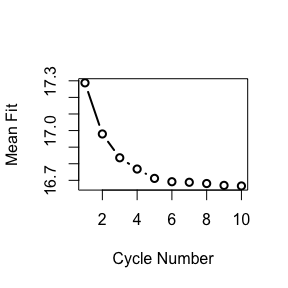The convergence of the arclength of the test information curve is also important to display since it tells us how much information is being tested for this set of examinees. cycleno <- 1:ncycle plot(cycleno, arclengthvec[cycleno], type="b", lwd=2, xlab="Cycle Number", ylab="Arclength")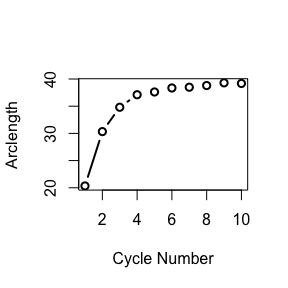This plot shows a nice exponential-like decline in the average fitting criterion meanHsave over 10 iterations. It does look like we could derive a small benefit from a few more iterations, but the changes in what we estimate using super precision in the minimization will be too small to be of any practical interest. The arc length of the information curve increases at each cycle as more and more information captured by the fit to the choice data. ### Setting up the parList objects to be used in further analyses and displays Here we choose to use results for the last cycle: Math_parListi <- parList[[ncycle]] The list object Math_parListi contains a large number of objects, but in our exploration of results, we will only need these results: WfdList <- Math_parListi$WfdList
theta      <- Math_parListi$theta Qvec <- Math_parListi$Qvec
Hval       <- Math_parListi$Hval DHval <- Math_parListi$DHval
D2Hval     <- Math_parListi$D2Hval binctr <- Math_parListi$binctr
indfine    <- seq(0,100,len=101)
• WfdList: List object of length n that contains information about the surprisal and probability curves
• theta: A vector of length N of values of what we call “score index”, which are real numbers between 0 and 100. These values are used for computing various score values such as expected sum scores.
• Qvec: Values within the range 0 to 100 below which these percentages of examinees fall: 5, 25, 50, 75 and 95.
• binctr: The surprisal curves are estimated by first binning the values in vector theta and then using the surprisal values within each bin. binctr contains the values at the centres of the bins.

### Check the score index for each fitting curve for being the global miniimum

Experience shows that in about 10 % of the cases the analysis locates the score index value theta at a minimum point along the fitting curve that is not the lowest point on the curve. We now check each examinee’s curve, and where the global minimum has not been identified, we do so by identifying the global minimum using a fine mesh of 101 values of theta. The revised value of theta is a location on this mesh, and objects Hval, DHval and D2Hval are also changed to their values at this point.

thetacheck  <- thetasearch(WfdList, U, theta, Hval, DHval, D2Hval)
theta       <- thetacheck$theta Hval <- thetacheck$Hval
DHval       <- thetacheck$DHval D2Hval <- thetacheck$D2Hval
changeindex <- thetacheck$changeindex print(paste("The number of altered theta values is", length(changeindex))) #  "The number of altered theta values is 183" Object changeindex is a vector containing the the indices of the examinees for whom the values have been altered. ### Compute objects needed for plotting as a function of arc length or information First we compute the quantities that we will need and same them in a named list: Math_infoList <- TestGardener::theta2arclen(theta, Qvec, WfdList, binctr) Then we retrieve these quantities: arclength <- Math_infoList$arclength
arclengthvec  <- Math_infoList$arclengthvec arclengthfd <- Math_infoList$arclengthfd
theta_al      <- Math_infoList$theta_al thetafine_al <- Math_infoList$thetafine.rng
Qvec_al       <- Math_infoList$Qvec_al binctr_al <- Math_infoList$binctr_al
Wfd_info      <- Math_infoList$Wfd_info Wdim <- Math_infoList$Wdim

### Plot surprisal curves for test questions over both the score index and arc length

Well, here we just plot the probability and surprisal curves for just the first question as an illustration. If argument plotindex is omitted, curves for all questions would be plotted. The correct answer curves for testing data are plotted as thick blue lines for highlighting, but for rating scale questions, this is omitted.

This plot plots probability and surprisal curves as a function of the score index theta:

indfine <- seq(0,100,len=101)
TestGardener::ICC.plot(indfine, WfdList, Math_dataList, Qvec, binctr,
data_point=TRUE, plotType=c("P", "W"), Wrng=c(0,3), plotindex=1)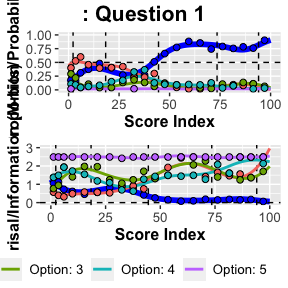Let’s make a few observations on what we see in these two plots.

When probability goes up to one, surprise declines to zero, as we would expect. The heavy blue curves show how the correct answer changes, and we are not surprised when top examinees with score index values close to 100 choose this answer. The purple and nearly straight curve is for the choice of not answering the question, and the probability of this is evidently nearly zero and the surprise is near three 5-bits, which is roughly equal to the surprise of tossing about 7 consecutive heads using a coin. (We convert 5-bits into 2-bits by multiplying 3 5-bits by 2.32, the value of the logarithm to the base 2 of 5.)

We see, too, that the right answer curve changes in a somewhat stepwise fashion, with two plateaus. In the first or left-most plateau we see that option 1 is chosen about as often as the right answer, and option 4 also appeals to these examinees. At about the 40% level, there is a sharp increase in probability because examinees at this level know that option 4 is not a candidate. The second smaller plateau happens because option 3 has some appeal to examinees near the 75% level.

It is the speed of an increase or decrease in the surprisal curve that is the fundamental signal that an examinee should be boosted up and dragged down, respectively, from a given position. The sharp increase in surprise for option 4 at the 40% level signals that an examinee in that zone should be increased. Of course the examinee’s final actual position will depend, not only on the five surprisal curves shown here, but also on those for the remaining 23 questions.

We call the rate of increase or decrease the “sensitivity” of an option. We have a specific plot for display this directly below.

The dots in the plot are the surprisal values for examinees in each of the 20 bins used in this analysis. The points are on the whole close their corresponding curves, suggesting that 1000 examinees gives us a pretty fair idea of the shape of a surprisal or probability curve.

Now let’s change the abscissa from the score index to the arclength or information index. We do this because arclength doesn’t change if we transform the score index in some way, and also arclength along the information curve has the extremely important metric property that a difference in arc lengths means exactly the same thing everywhere along the arc length interval.

indfine <- seq(0,100,len=101)
TestGardener::ICC.plot(arclengthvec, WfdList, Math_dataList, Qvec_al, binctr_al,
data_point=TRUE, plotType=c("P", "W"), Wrng=c(0,3), plotindex=1)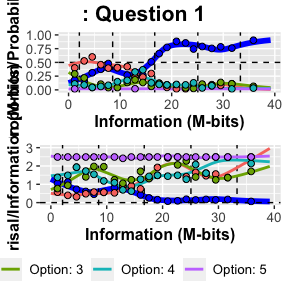What has changed? We see that now the high performance right sidie of the plot has been spread out. This provides us with more information and more accuracy in assessing performance in this important performance level.

## Plot the probability density of the score index and of arc length

The density of the percentile ranks that we initially used will no longer be a flat line.
This is because examinees tend to cluster at various score levels, no matter how the score is defined. Certain groups of items will tend to be correctly answered by the middle performance folks and other by only the top performers. Among the weakest examinees, there will still be a subset of questions that they can handle. We want to see these clusters emerge.

TestGardener::density_plot(theta, c(0,100), Qvec, xlabstr="Score index",
titlestr="Theta Density", scrnbasis=15)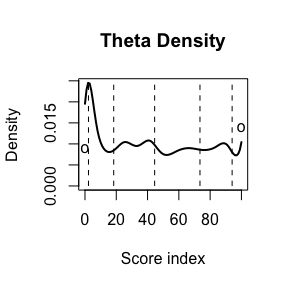In fact, there are four distinct clusters of score index values below the 75% level. Within each of these clusters there are strong similarities in examinee’s choice patterns, whether right or wrong. We have only plotted score indices which are away from the two boundaries, because there are significant tendencies to have estimated score index values at 0 and 100.

TestGardener::density_plot(theta_al, c(0,arclength), Qvec_al, xlabstr="Arclength",
titlestr="Arc length Density",  scrnbasis=15)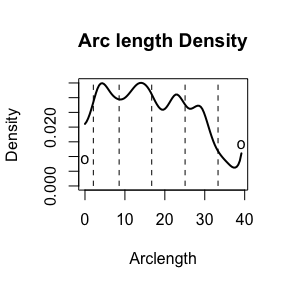## Compute expected test scores and plot their density function for all examinees

The expected score for an examinee are determined by his value theta for the question and by the score values assigned by the test designer to each option. We use a plotting interval defined by the range of the sum scores, but as a rule the density of expected scores is positive only over a shorter interval.

indfine <- seq(0,100,len=101)
mufine <- TestGardener::testscore(indfine, WfdList, optList)
TestGardener::mu.plot(mufine, Math_dataList$scrrng, titlestr)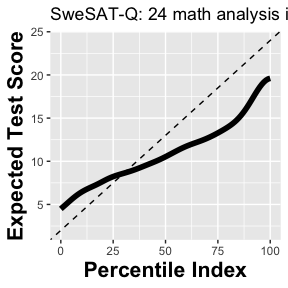Note that the bottom 5% examinees get on the average sum scores of about 5, which is 20% of the largest possible score of 24. This is because, even though they know nearly nothing about math, they can still score right often by just guessing or randomly choosing. But at the top end, the best examinees lose heavily in average score terms. There are no average scores above 21, even though there are plenty of examinees with score indices of nearly or exactly 100. This effect is due to the fact that a few questions are faulty in the sense of not getting close to probability one or surprisal 0 at score index 100. We found, for example, that one question did not have any answer that could be judged correct by serious mathematical standards. And another question seemed to suffer from some teachers having overlooked covering the topic of “percent increase.” It is typical that lower expected test scores are above the diagonal line and higher ones below. At the lower or left end, expected test scores are too high because of the possibility of getting question right by guessing. At the upper or right end the scores are below the line because of questions that fail to reach probability one for the right answer for even the strongest examinees. The process of computing an expected score compresses the range of scores relative to that of the observed test scores. ## Compute the arc length of the test effort curve and plot the curve We have 412 curves simultaneously changing location simultaneously in both probability and surprisal continua as the score index moves from 0 to 100. We can’t visual such a thing, but we are right to think of this as a point moving along a curve in these two high dimensional spaces. In principle this curve has twists and turns in it, but we show below that they are not nearly as complex as one might imagine. What we can study, and use in many ways, is the distance within the curve from its beginning to either any fixed point along it, or to its end. Thße curve is made especially useful because it can be shown that any smooth warping of the score index continuum will have no impact on the shape of this curve. Distance along the curve can be measured in bits, and a fixed change in bits has the same meaning at every point in the curve. The bit is a measure information, and we call this curve the test information curve. The next analysis and plot displays the length of the curve and how it changes relative to the score index theta associated with any point on the curve. print(paste("Arc length =", round(arclength,2))) #  "Arc length = 39.18" TestGardener::ArcLength.plot(arclength, arclengthvec, titlestr)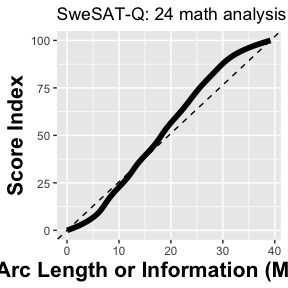The relationship is quite linear, except for some curvature near 0 and 100. We can say of the top examinees that they acquire nearly 90 2-bits of information represented in the test. That is, the probability of aceing this test by chance alone is that of tossing 90 heads in a row. (We convert 5-bits into 2-bits by multiplying 39 by 2.32]) ## Display test information curve projected into its first two principal components The test information curve is in principle an object of dimension Wdim, but in most cases almost all of its shape can be seen in either two dimensions or three. Here we display it in two dimensions as defined by a functional principal component analysis. Result <- TestGardener::Wpca.plot(arclength, WfdList, Math_dataList$Wdim, titlestr=titlestr)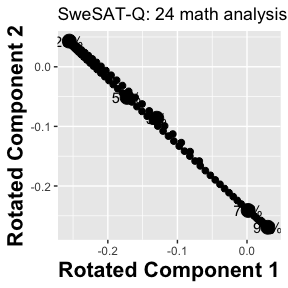The change in direction of this curve between the 25% and 50% marker points indicates that the information acquired initially is qualitatively different than that mastered at the upper end of the curve. This seems obvious, since math moves from the concrete to the abstract over a secondary school program.

## Investigate the status of score index estimate by plotting H(theta) and its derivatives

An optimal fitting criterion for modelling test data should have these features: (1) at the optimum value of theta fit values at neighbouring values should be larger than the optimum; (2) the first derivative of the fitting criterion should be zero or very nearly so, and (3) the second derivative should have a positive value.

But one should not automatically assume that there is a single unique best score index value that exists for an examinee or a ratings scale respondent. It’s not at all rare that some sets of data display more than one minimum. After all, a person can know some portion of the information at an expert level but be terribly weak for other types of information. By tradition we don’t like to tell people that there are two or more right answers to the question, “How much do you know?” But the reality is otherwise, and especially when the amount of data available is modest.

If an estimated score index seems inconsistent with the sum score value or is otherwise suspicious, the function Hfuns.plot() allows us to explore the shape of the fitting function H(theta) as well as that of its second derivative.

Here we produce these plots for the first examinee.

TestGardener::Hfuns.plot(theta, WfdList, U, plotindex=1)`